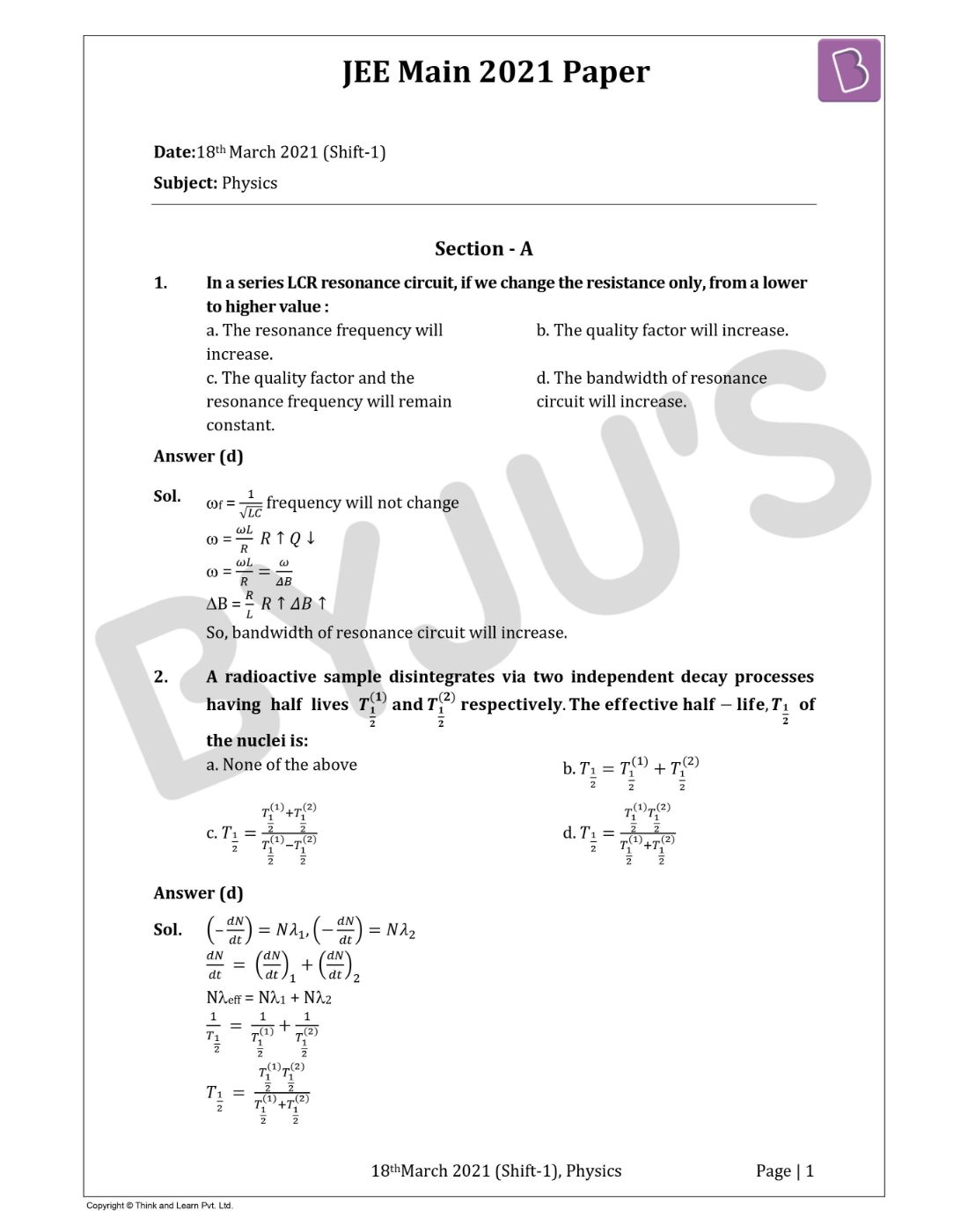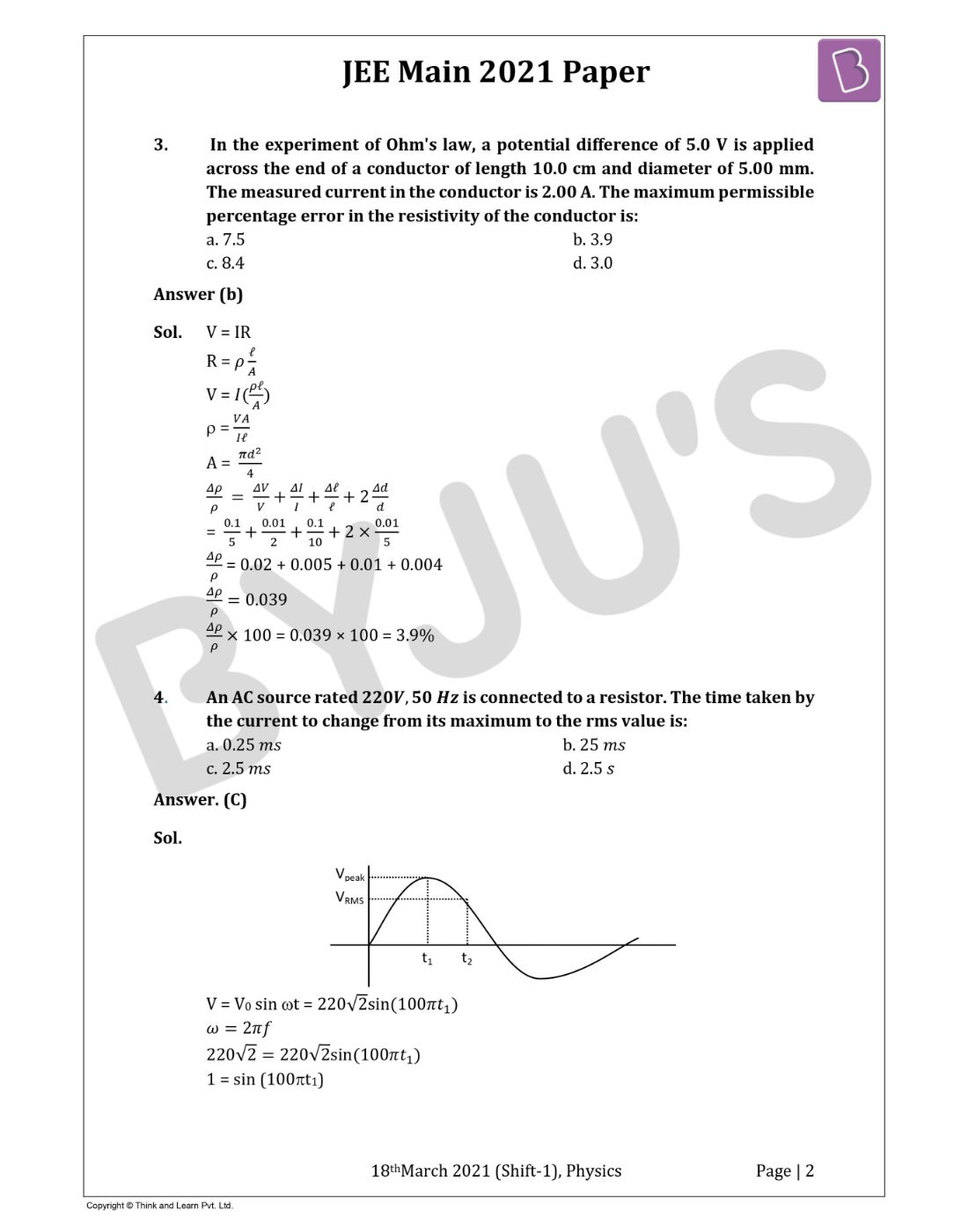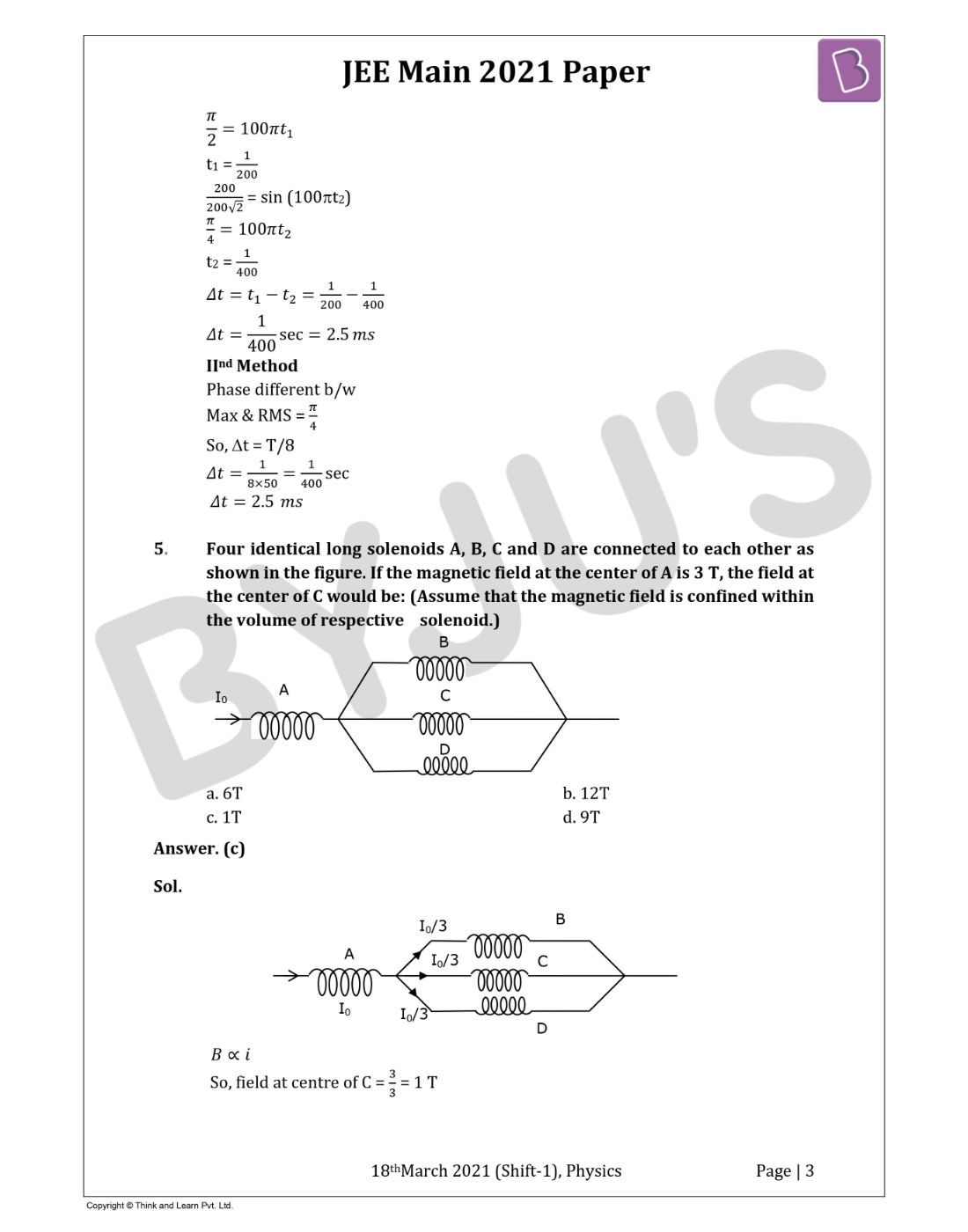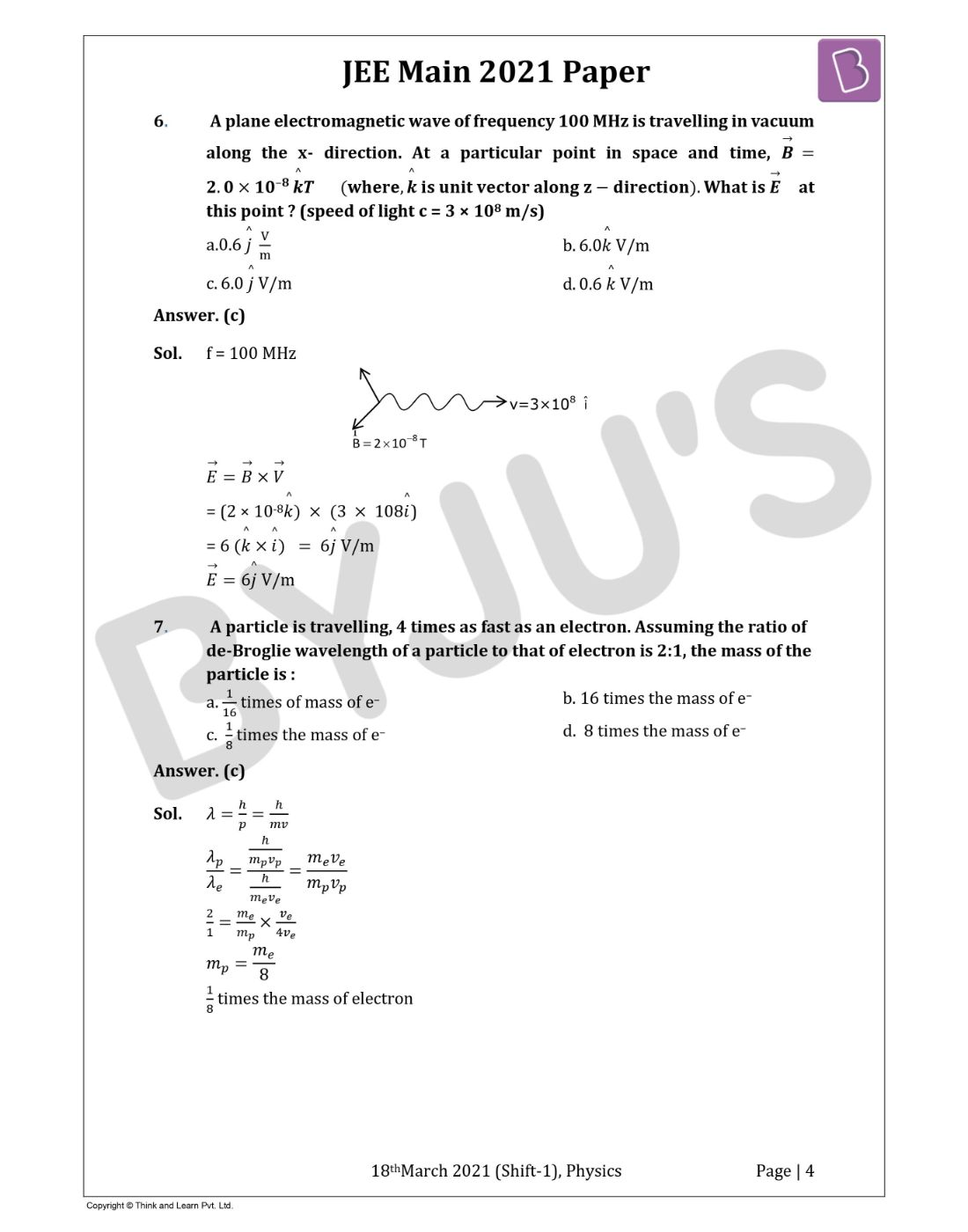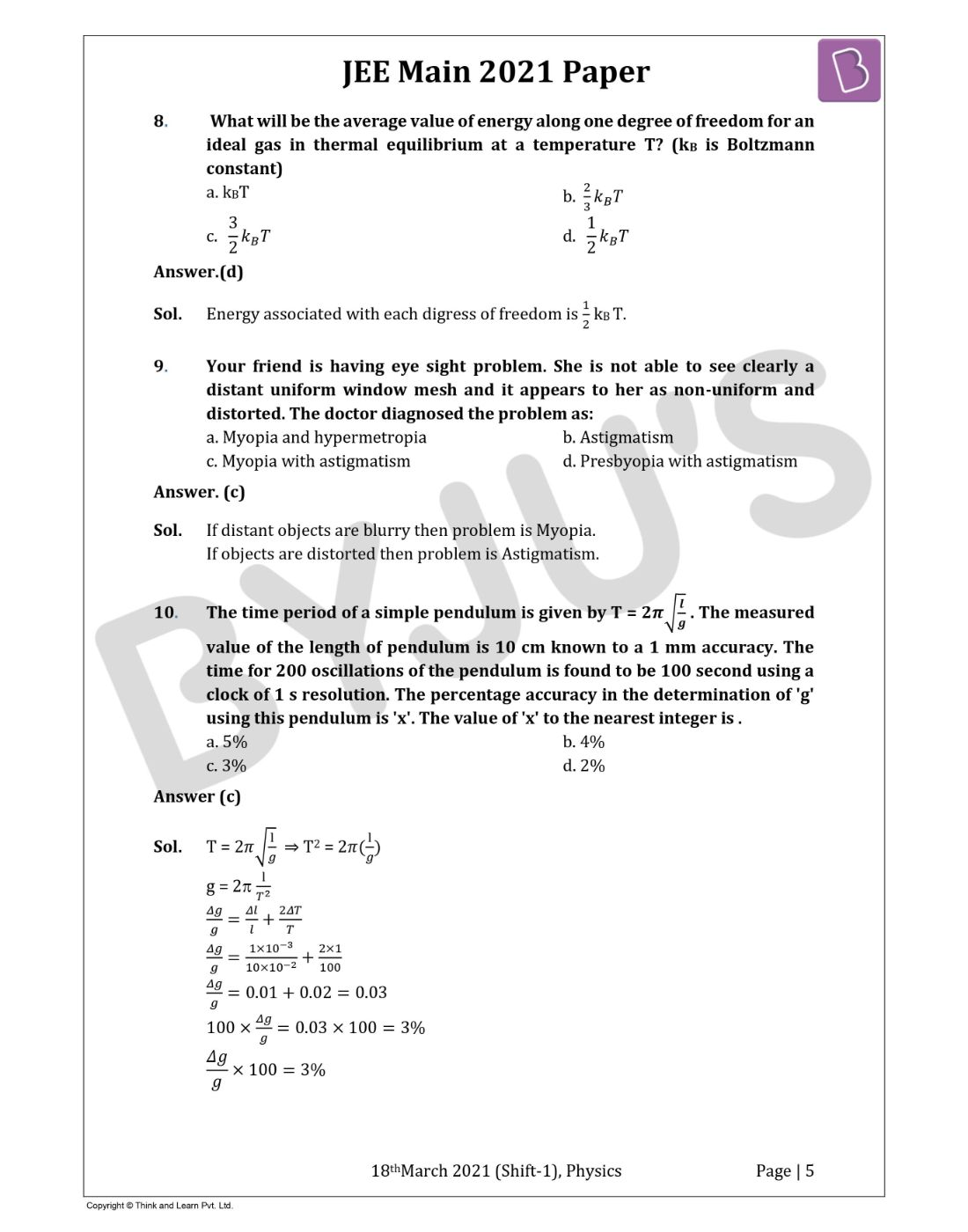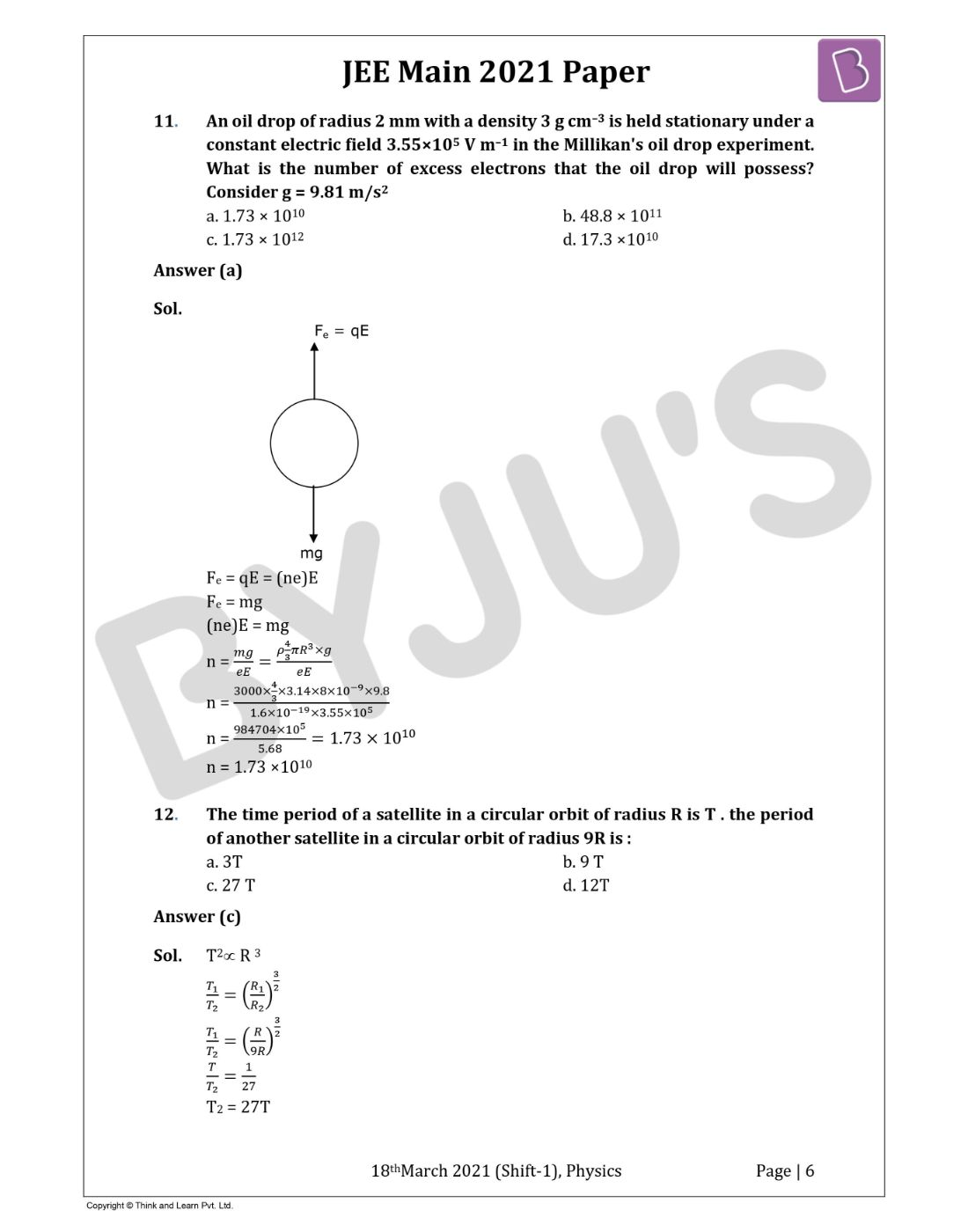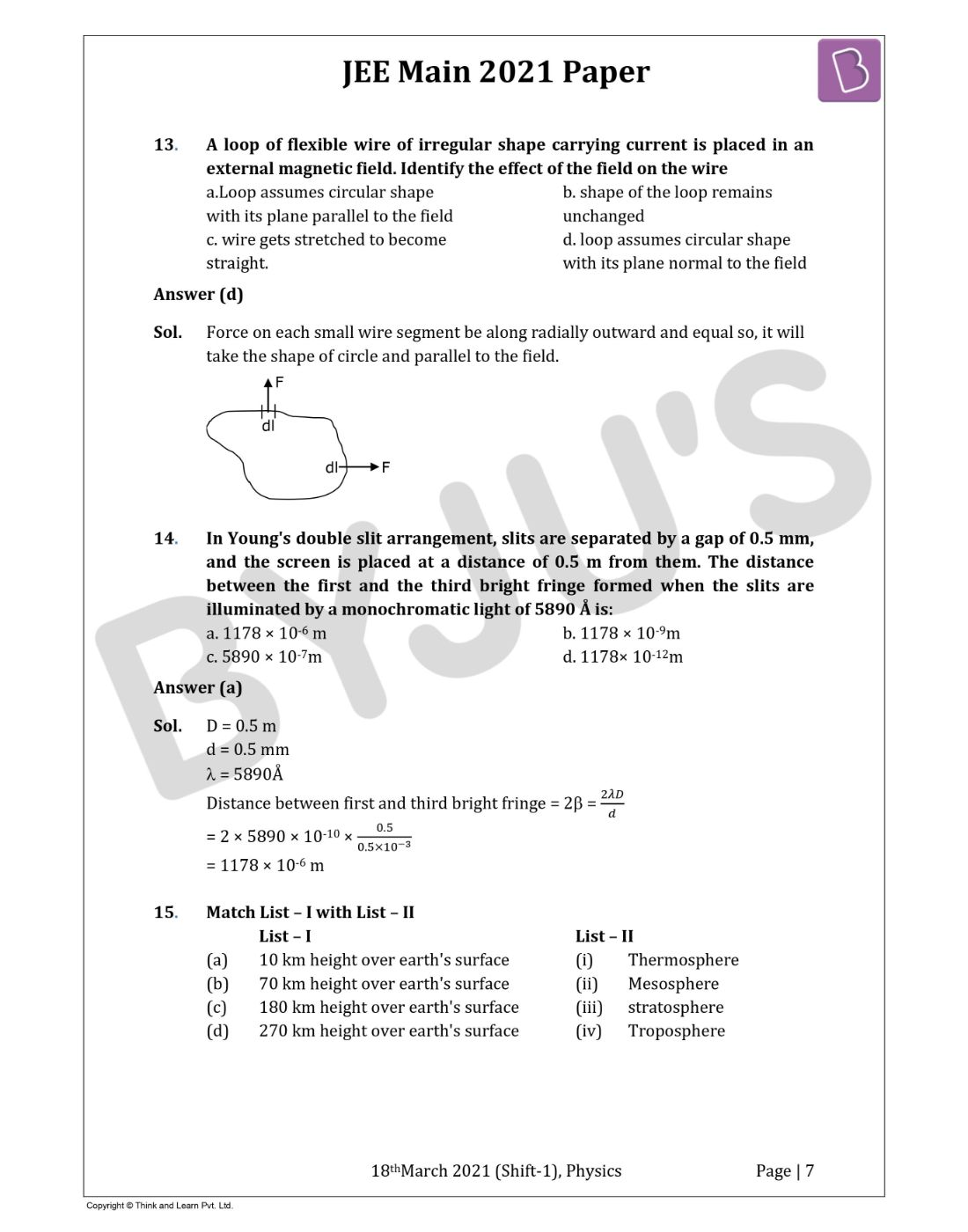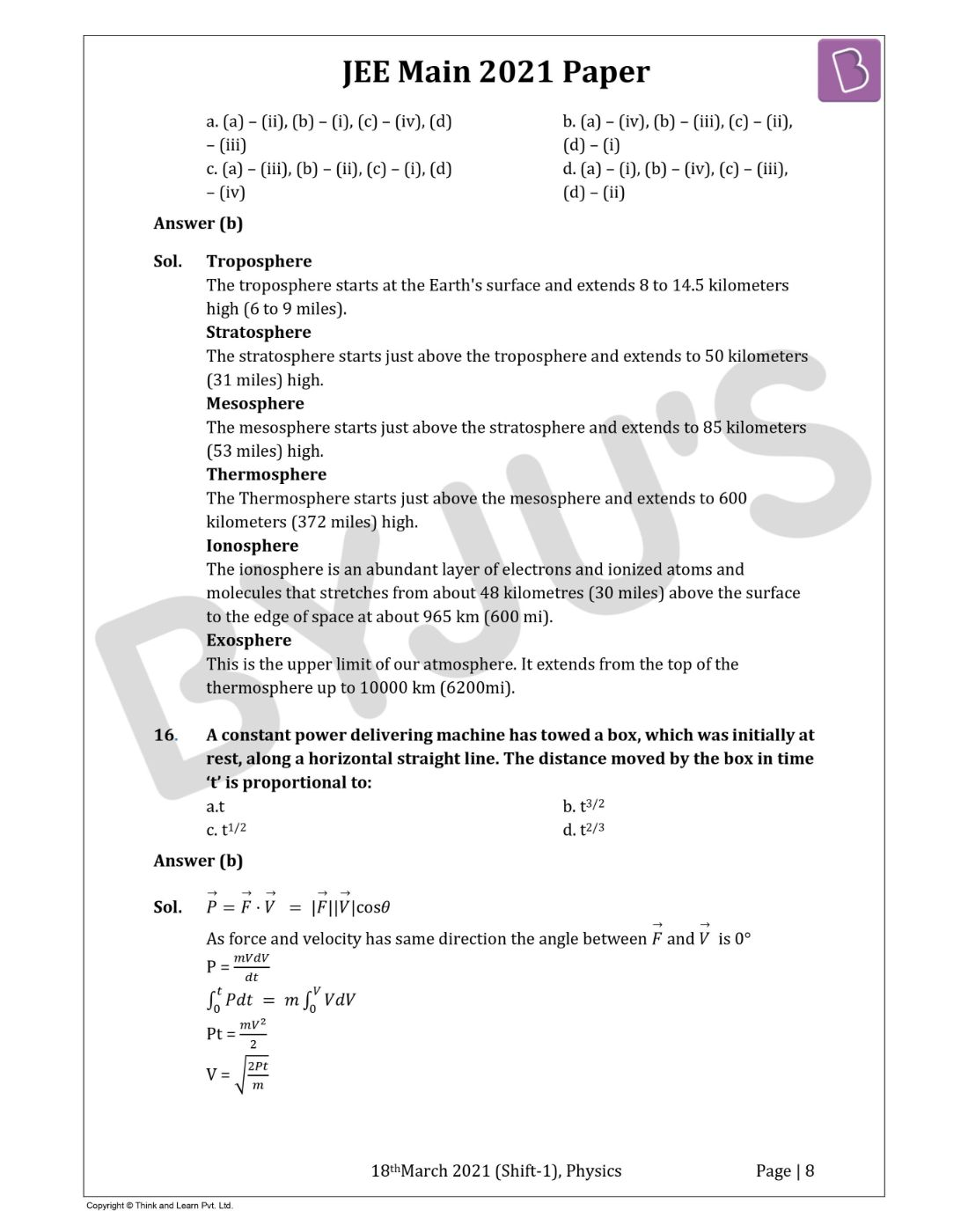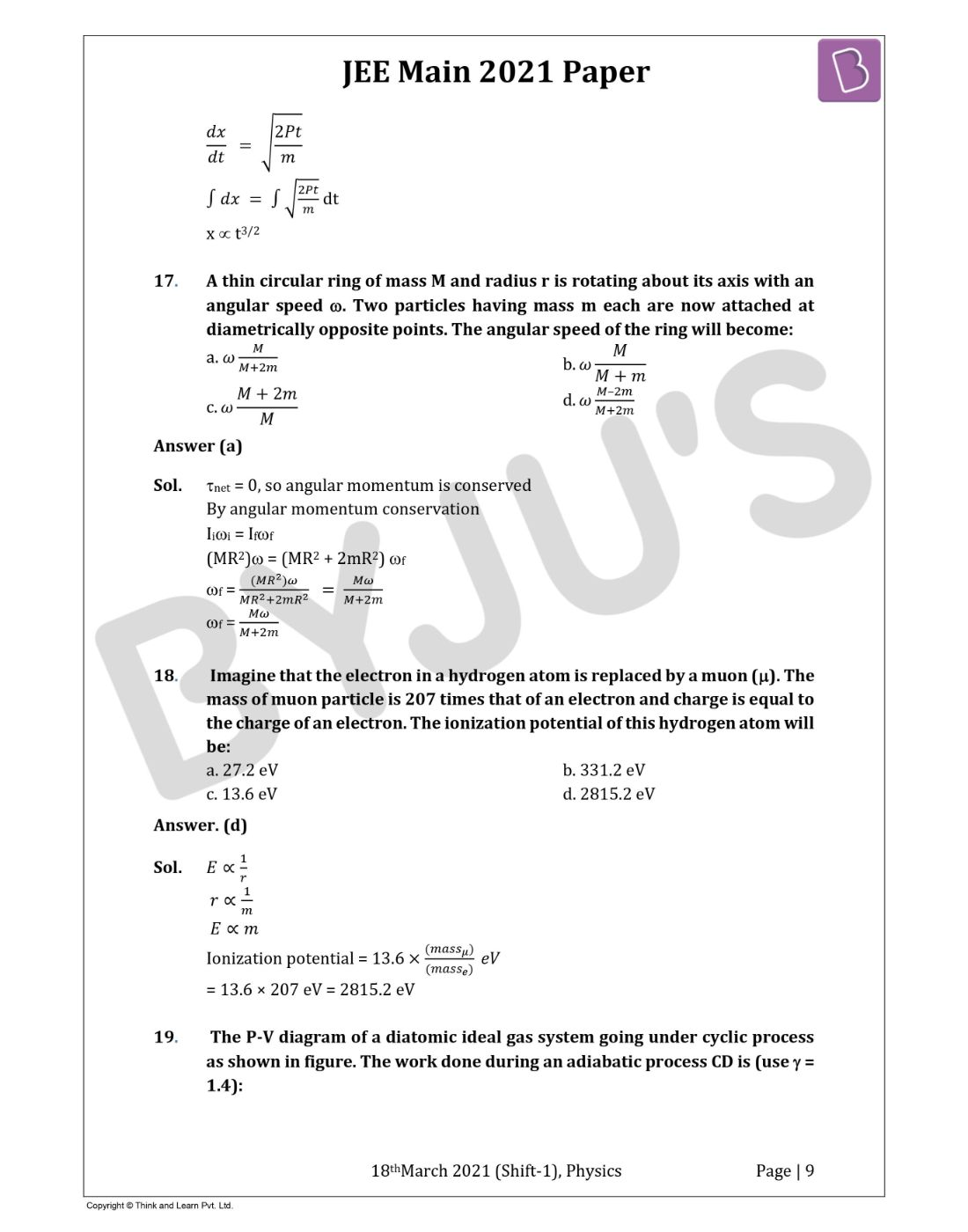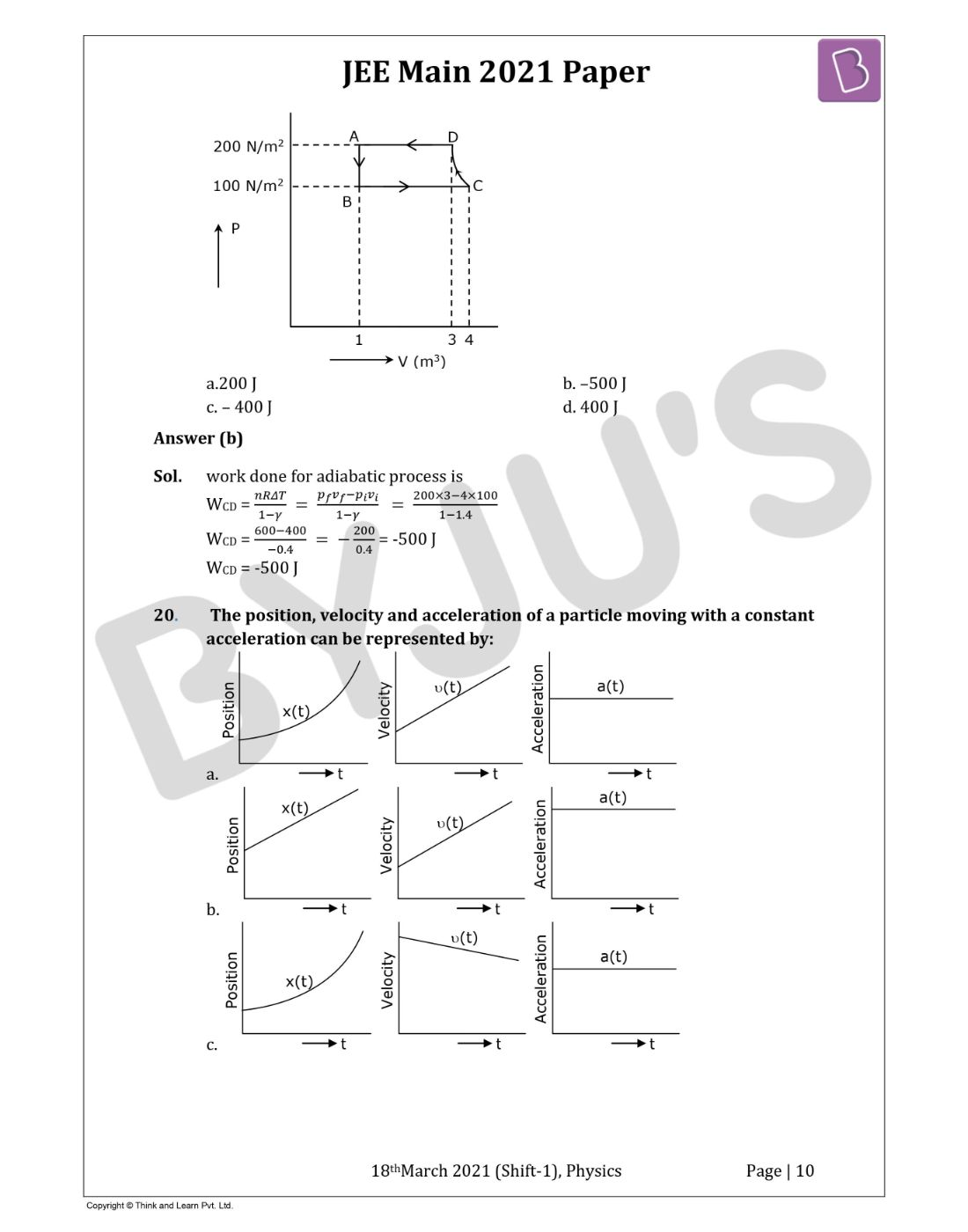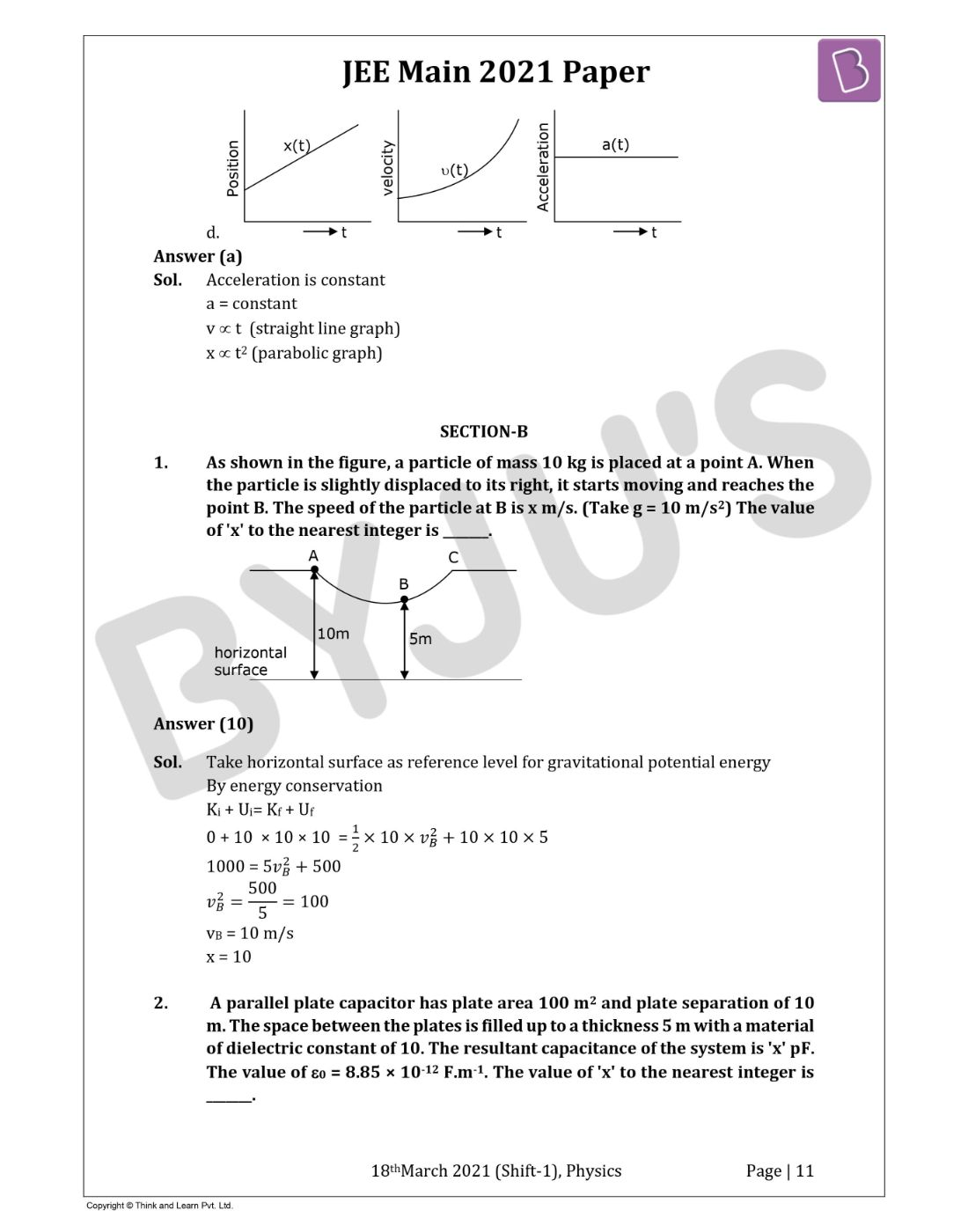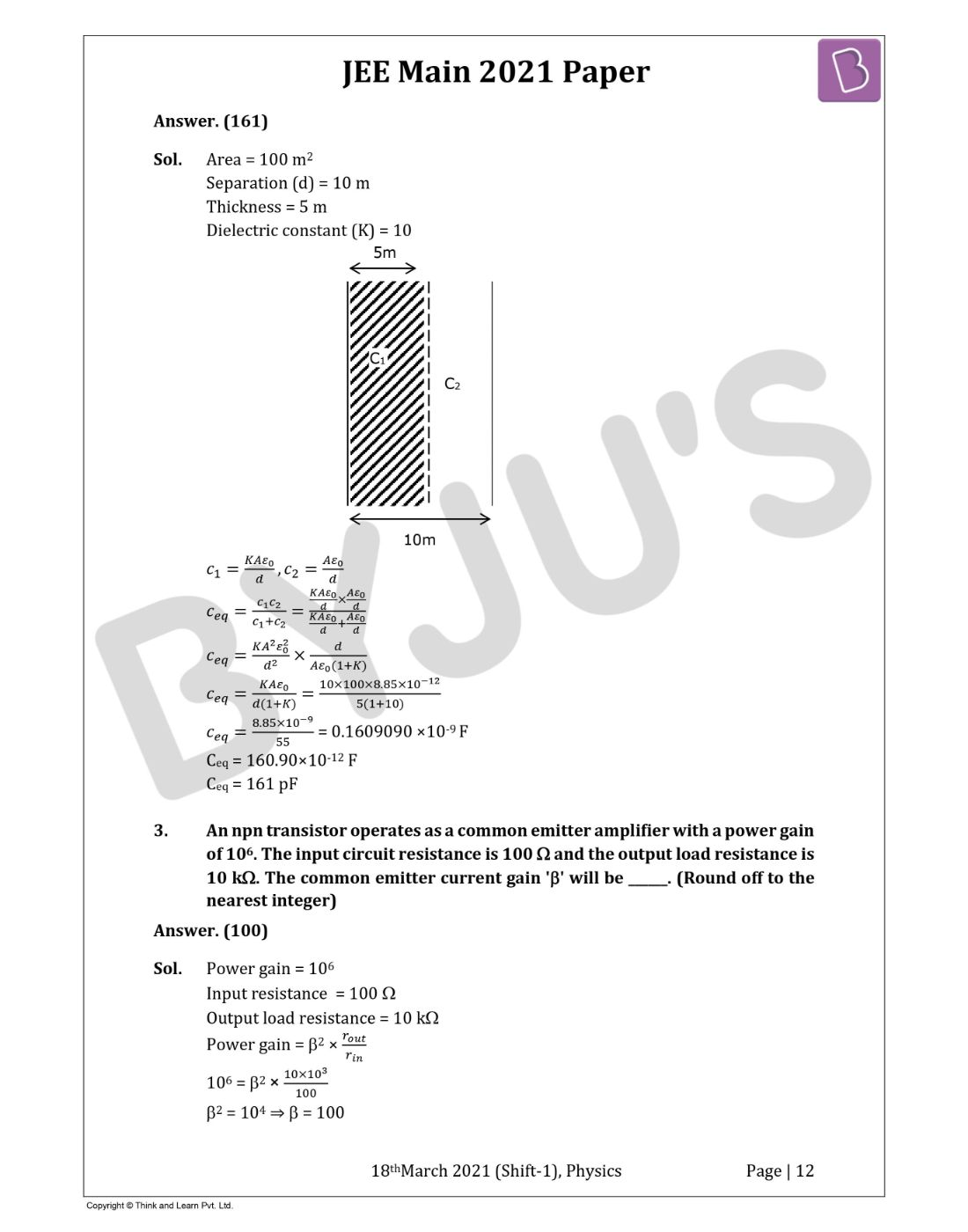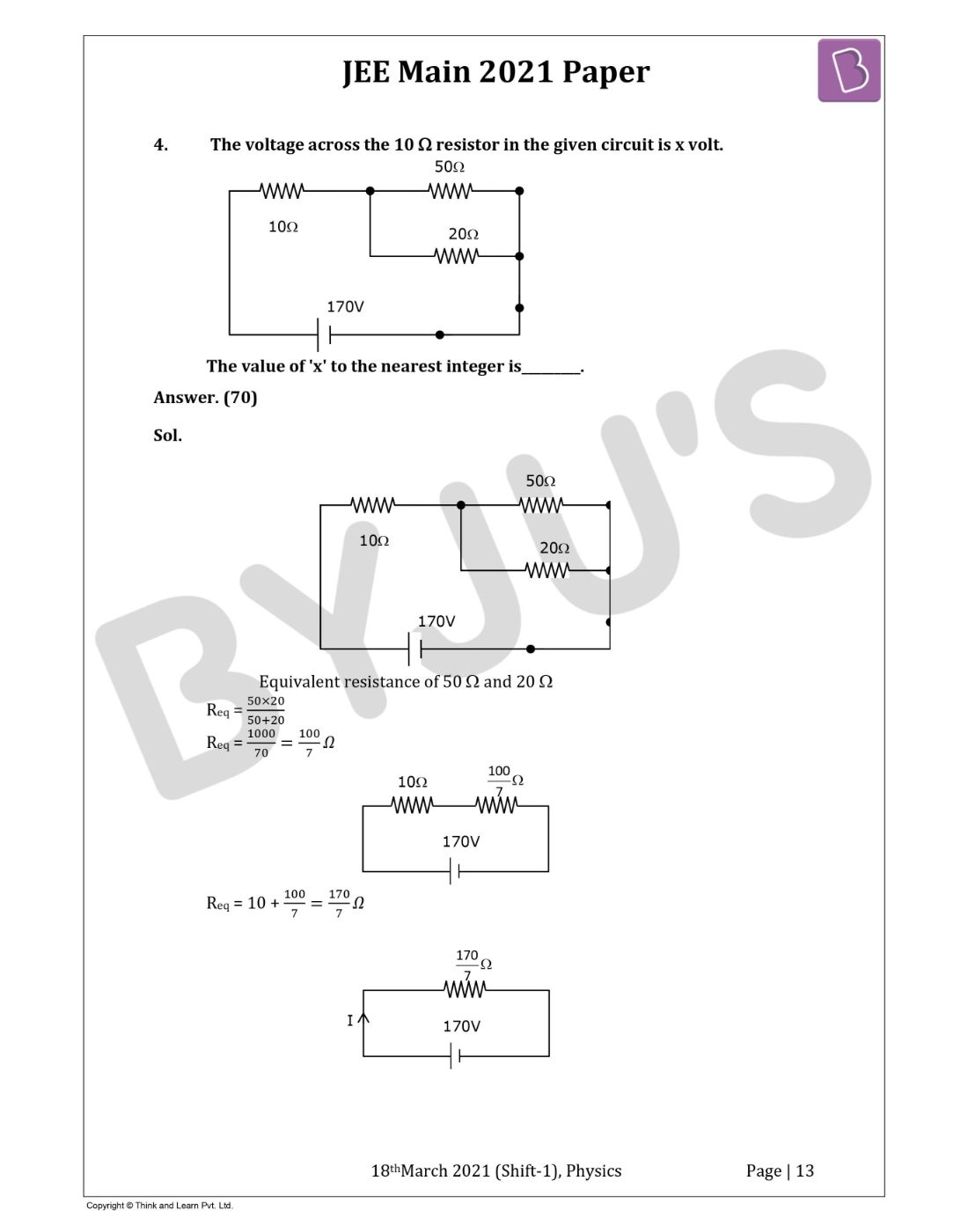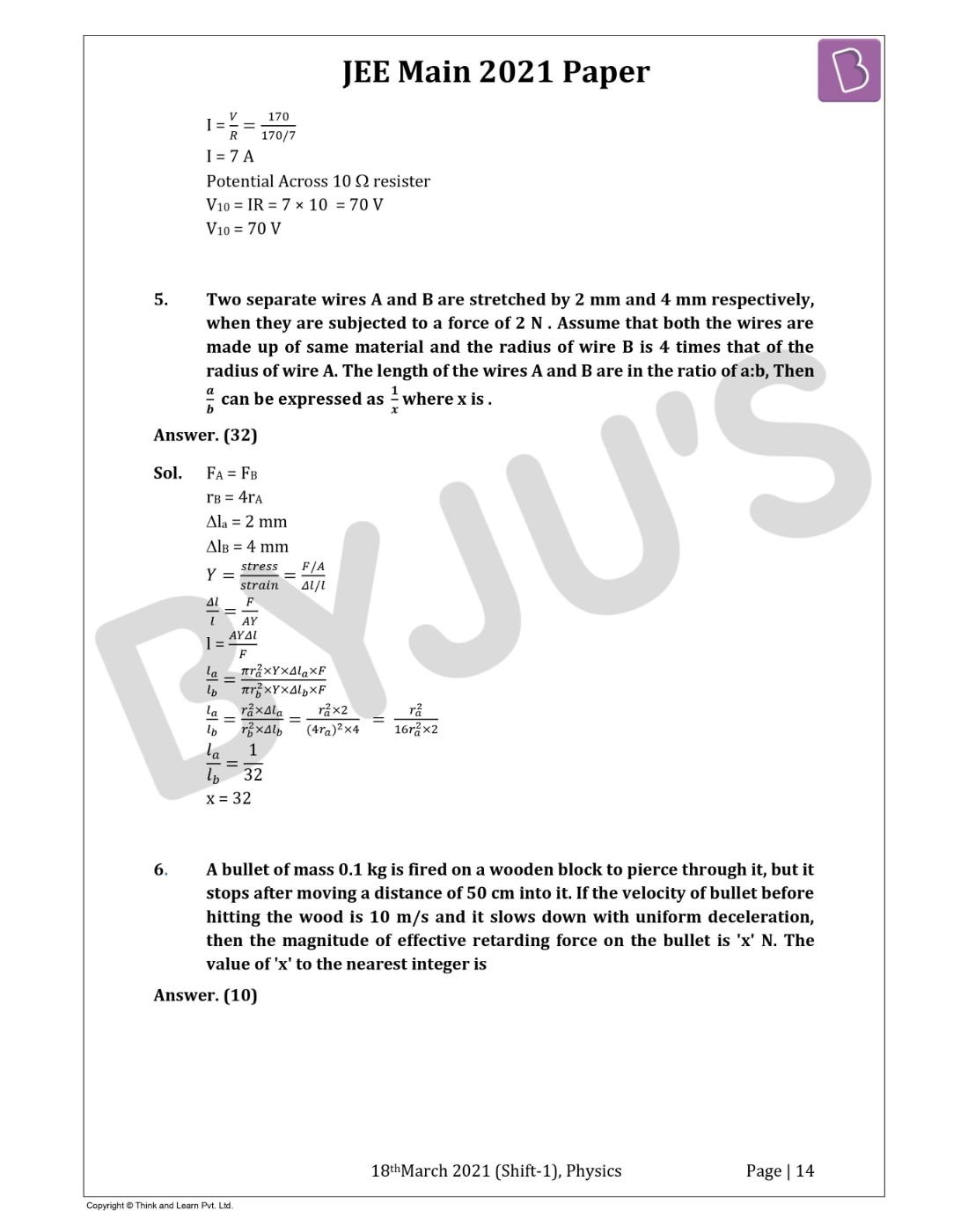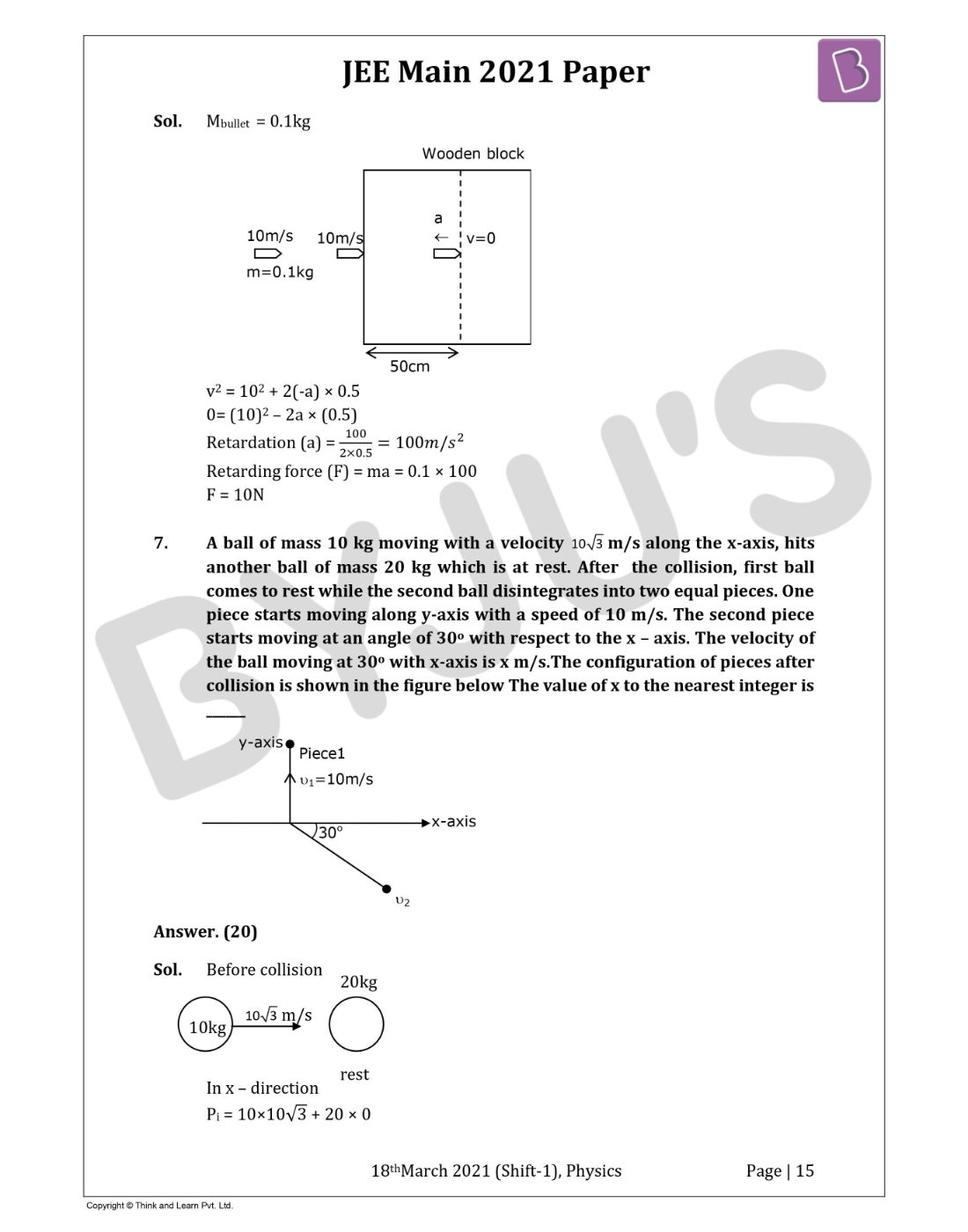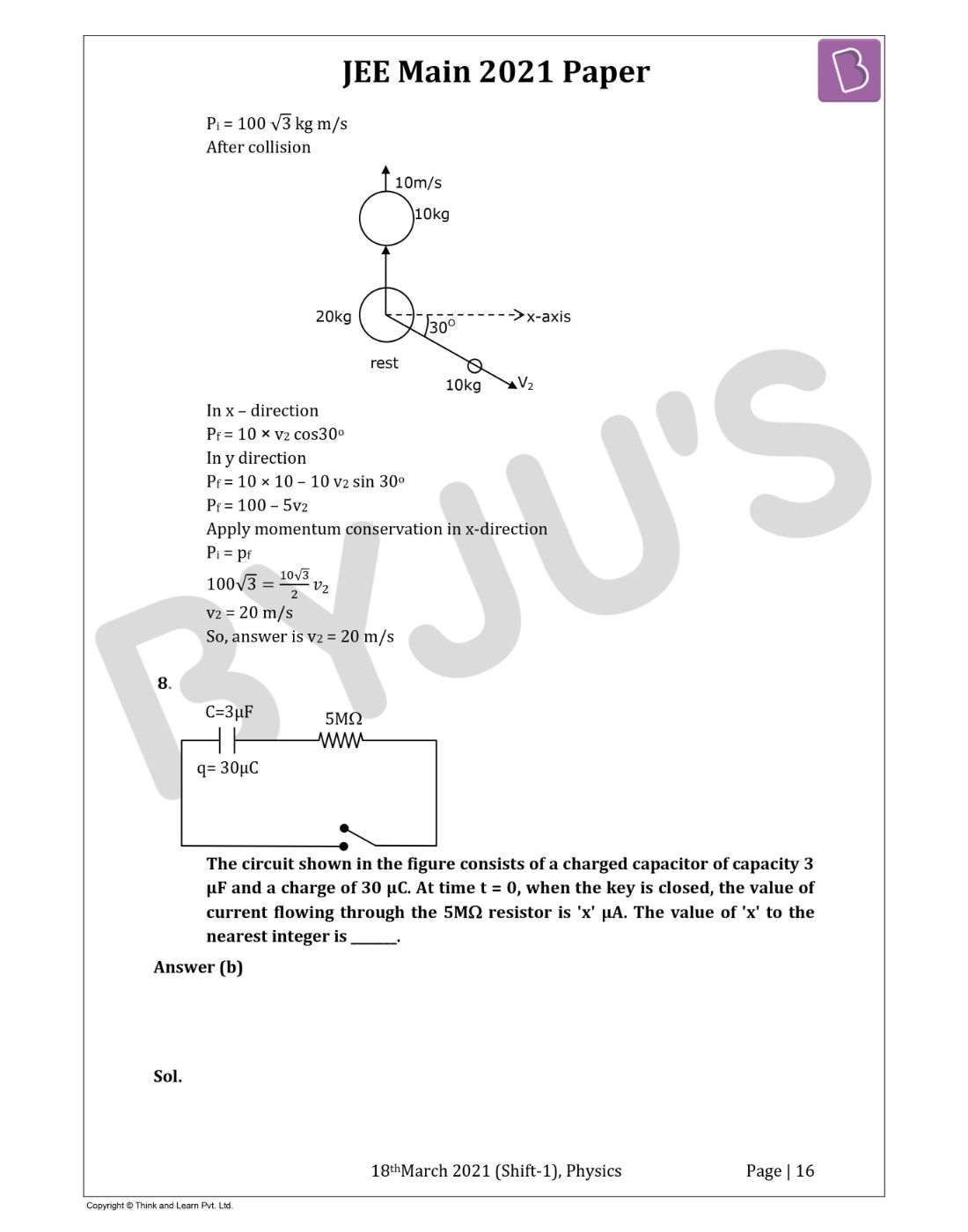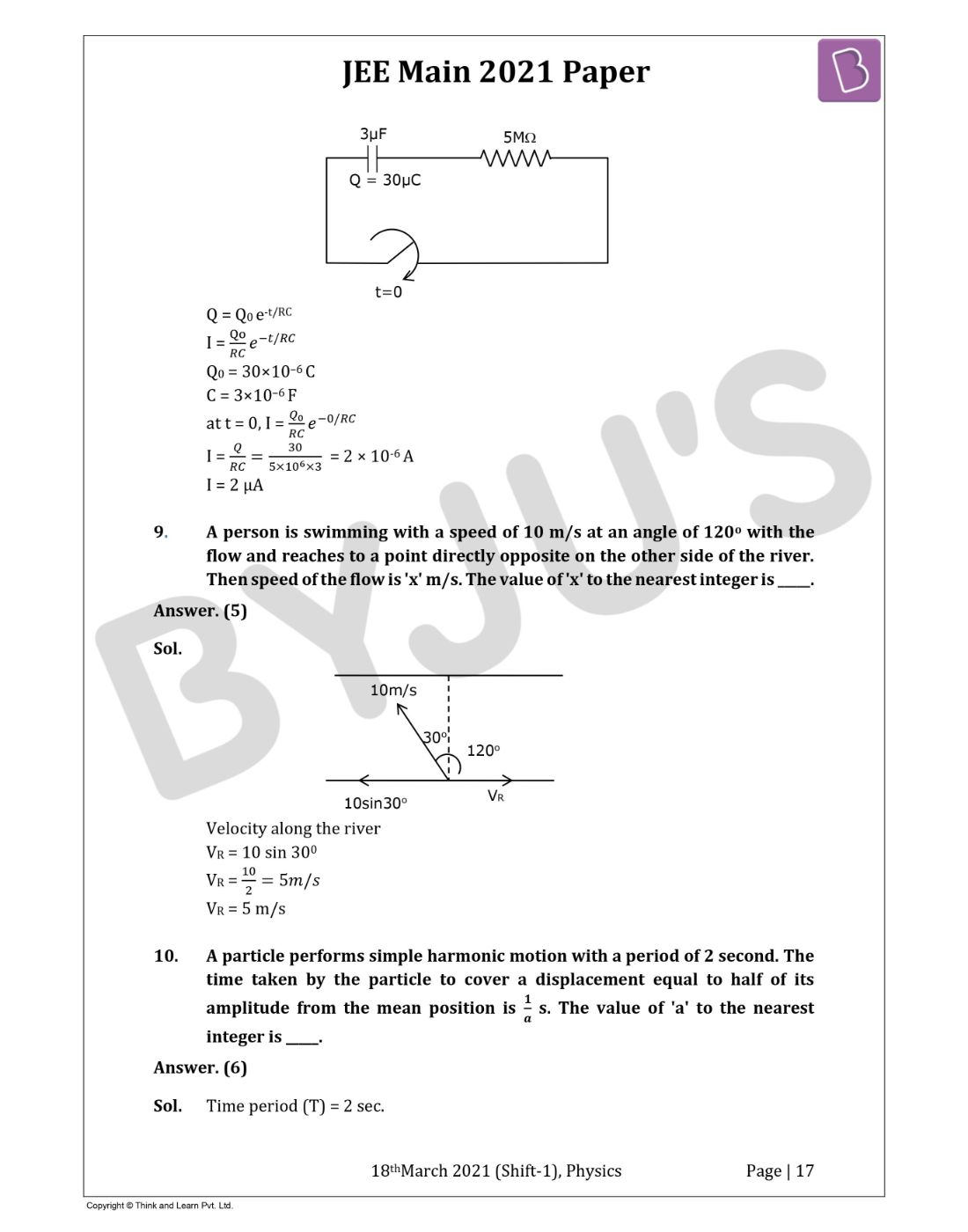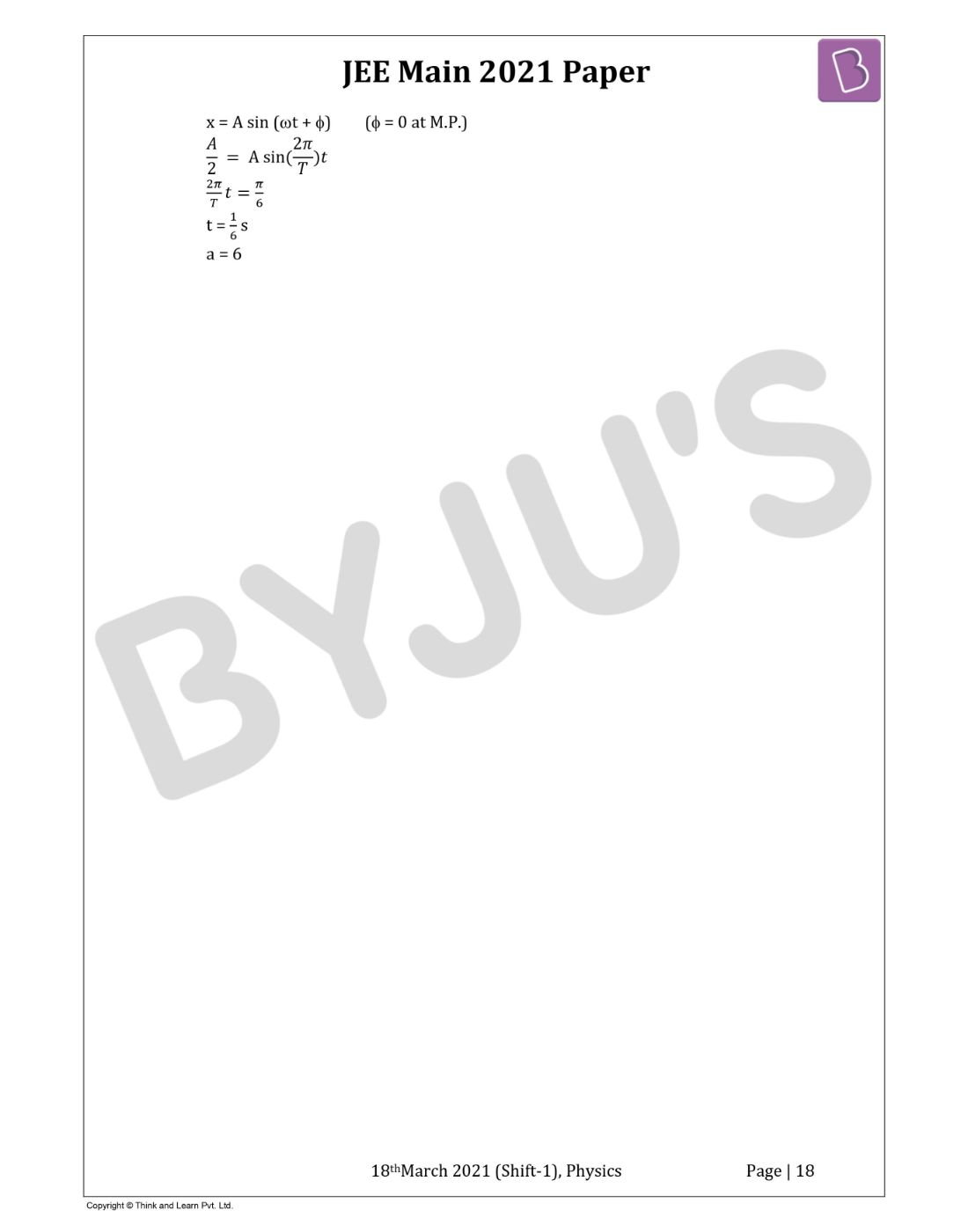Gauge your potential with Aakash BYJU'S JEE Main'23 Mock Test Gauge your potential with Aakash BYJU'S JEE Main'23 Mock Test

# JEE Main 2021 March 18 – Shift 1 Physics Question Paper with Solutions

JEE Main 2021 March 18th shift 1 Physics question paper with solutions are given on this page. Practising JEE Main 2021 question papers will help students to score more marks for the upcoming JEE Main exams. These solutions are prepared by the expert team at BYJU’S. Students can also easily download the PDF format of the solutions. These solutions will help JEE aspirants to get an idea of the difficulty level and marking scheme of the exam.
JEE Main 2021 March 18th Shift 1 Physics Question Paper

Section – A

Question 1. In a series LCR resonance circuit, if we change the resistance only, from a lower to higher value :

a. The resonance frequency will increase
b. The quality factor will increase
c. The quality factor and the resonance frequency will remain constant
d. The bandwidth of the resonance circuit will increase

$$\begin{array}{l}\omega _{f}= \frac{1}{\sqrt{LC}}\end{array}$$
frequency will not change

$$\begin{array}{l}\begin{array}{l} Q=\frac{\omega L}{R} (R \uparrow Q \downarrow) \\ Q =\frac{\omega L}{R}=\frac{\omega}{\Delta B} \\ \Delta \mathrm{B}=\frac{R}{L} (R \uparrow \Delta B \uparrow) \end{array}\end{array}$$

So, the bandwidth of the resonance circuit will increase.

Question 2. A radioactive sample disintegrates via two independent decay processes having half-livesT1/21 and T1/22respectively. The effective half-life, T1/2 of the nuclei is:

a. None of the above
b.

$$\begin{array}{l}T_{1/2}= T_{1/2}^{1}+T_{1/2}^{2}\end{array}$$

c.
$$\begin{array}{l}T_{1/2}= \frac{T_{1/2}^{1}+T_{1/2}^{2}}{T_{1/2}^{1}-T_{1/2}^{2}}\end{array}$$

d.
$$\begin{array}{l}T_{1/2}= \frac{T_{1/2}^{1}T_{1/2}^{2}}{T_{1/2}^{1}+T_{1/2}^{2}}\end{array}$$

$$\begin{array}{l}\left ( -\frac{dN}{dt} \right )= N\lambda _{1}\end{array}$$
$$\begin{array}{l}\left ( -\frac{dN}{dt} \right )= N\lambda _{2}\end{array}$$
$$\begin{array}{l}\left ( \frac{dN}{dt} \right )= \left ( \frac{dN}{dt} \right )_{1}+\left ( \frac{dN}{dt} \right )_{2}\end{array}$$
$$\begin{array}{l}N\lambda _{eff}=N\lambda _{1}+N\lambda _{2}\end{array}$$
$$\begin{array}{l}\frac{1}{T_{1/2}}=\frac{1}{T_{1/2}^{1}}+\frac{1}{T_{1/2}^{2}}\end{array}$$
$$\begin{array}{l}T_{1/2}= \frac{T_{1/2}^{1}T_{1/2}^{2}}{T_{1/2}^{1}+T_{1/2}^{2}}\end{array}$$

Question 3. In the experiment of Ohm’s law, a potential difference of 5.0 V is applied across the end of a conductor of length 10.0 cm and diameter of 5.00 mm. The measured current in the conductor is 2.00 A. The maximum permissible percentage error in the resistivity of the conductor is:

a. 7.5
b. 3.9
c. 8.4
d. 3.0

V = IR

$$\begin{array}{l}R=\rho (\frac{l}{A})\end{array}$$
$$\begin{array}{l}V = I\left ( \frac{\rho l}{A} \right )\end{array}$$
$$\begin{array}{l}\rho =\frac{VA}{Il}\end{array}$$
$$\begin{array}{l}A = \frac{\pi d^{2}}{4}\end{array}$$
$$\begin{array}{l}\frac{\Delta \rho }{\rho }=\frac{\Delta V}{V}+\frac{\Delta I}{I}+\frac{\Delta I}{I}+2\frac{\Delta d}{d}\end{array}$$
$$\begin{array}{l}\frac{\Delta \rho }{\rho }=\frac{0.1}{5}+\frac{0.01}{2}+\frac{0.1}{10}+2\frac{0.01}{5}\end{array}$$
$$\begin{array}{l}\frac{\Delta \rho }{\rho }= 0.02 + 0.005 + 0.01 + 0.004\end{array}$$
$$\begin{array}{l}\frac{\Delta \rho }{\rho }= 0.039\end{array}$$
$$\begin{array}{l}\frac{\Delta \rho }{\rho }\times 100= 0.039\times 100 = 3.9%\end{array}$$

Question 4. An AC source rated 220V, 50 Hz is connected to a resistor. The time taken by the current to change from its maximum to the rms value is:

a. 0.25 ms
b. 25 ms
c. 2.5 ms
d. 2.5 s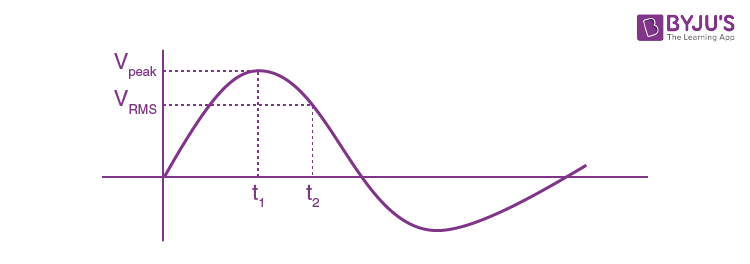$$\begin{array}{l}V=V_{0}sin\omega t= 220\sqrt{2}sin(100\pi t_{1})\end{array}$$
$$\begin{array}{l}\omega = 2\pi f\end{array}$$
$$\begin{array}{l}200\sqrt{2}=220\sqrt{2}sin(100\pi t_{1})\end{array}$$
$$\begin{array}{l}1=sin(100\pi t_{1})\end{array}$$
$$\begin{array}{l}\frac{\pi }{2}=100\pi t_{1}\end{array}$$
$$\begin{array}{l}t_{1}=\frac{1}{200}\end{array}$$
$$\begin{array}{l}\frac{200}{200\sqrt{2}}=sin(100\pi t_{2})\end{array}$$
$$\begin{array}{l}\frac{\pi }{4}=100\pi t_{2}\end{array}$$
$$\begin{array}{l}t_{2}=\frac{1}{400}\end{array}$$

Δt=t1– t2=(1/200) – (1/400)

Δt=(1/400) sec= 2.5 ms

IInd Method

Phase difference b/w

Max & RMS =𝜋/4

So, Δt = T/8

Δt=1/(8×50) =1/400sec

Δt= 2.5  ms

Question 5. Four identical long solenoids A, B, C and D are connected to each other as shown in the figure. If the magnetic field at the centre of A is 3 T, the field at the centre of C would be: (Assume that the magnetic field is confined within the volume of the respective solenoid.)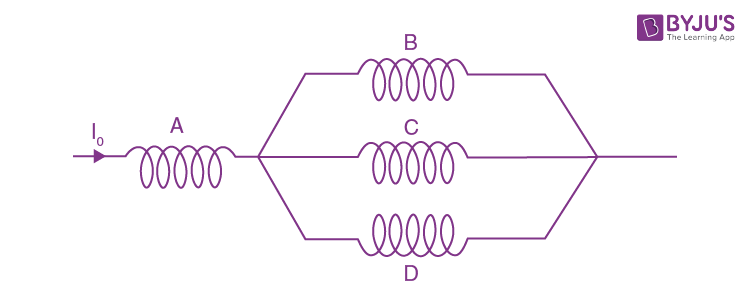a. 6T
b. 12T
c. 1T
d. 9T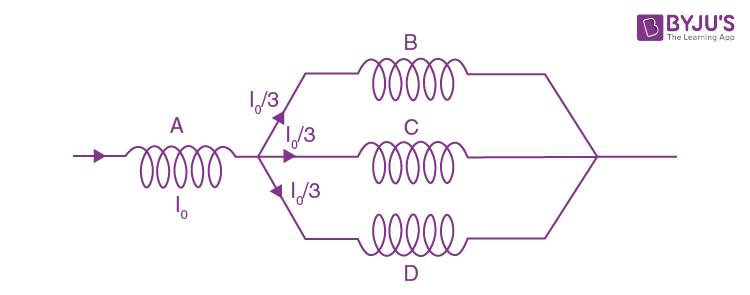B∝i

So, field at centre of C = 3/3 = 1 T

Question 6. A plane electromagnetic wave of frequency 100 MHz is travelling in a vacuum along the x-direction. At a particular point in space and time,

$$\begin{array}{l}\vec{B}=2.0 \times 10^{-8}kT\end{array}$$

(where

$$\begin{array}{l}\hat{k}\end{array}$$
is the unit vector along the z-direction). What is
$$\begin{array}{l}\vec{E}\end{array}$$
at this point? (speed of light c = 3 × 108 m/s)

a.

$$\begin{array}{l}0.6\hat{j}\frac{V}{m}\end{array}$$

b.
$$\begin{array}{l}6.0\hat{k}\frac{V}{m}\end{array}$$

c.
$$\begin{array}{l}6.0\hat{j}\frac{V}{m}\end{array}$$

d.
$$\begin{array}{l}0.6\hat{k}\frac{V}{m}\end{array}$$

f = 100 MHz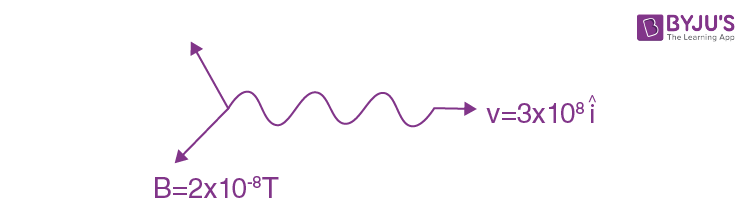$$\begin{array}{l}\vec{E}=\vec{B}\times \vec{V}\end{array}$$
$$\begin{array}{l}\vec{E}=2\times 10^{-8}k\times 3\times 10^{8}i\end{array}$$
$$\begin{array}{l}\vec{E}=6(\hat{k}\times \hat{i})=6\hat{j}V/m\end{array}$$

Question 7. A particle is travelling, 4 times as fast as an electron. Assuming the ratio of the de-Broglie wavelength of a particle to that of the electron is 2:1, the mass of the particle is :

a. 1/16 times of mass of e
b. 1/6 times the mass of e
c. 1/8 times the mass of e
d. 8 times the mass of e

$$\begin{array}{l}\lambda =\frac{h}{p}=\frac{h}{mv}\end{array}$$
$$\begin{array}{l}\frac{\lambda _{p}}{\lambda _{e}}=\frac{\frac{h}{m_{p}v_{p}}}{\frac{h}{m_{e}v_{e}}}=\frac{m_{e}v_{e}}{m_{p}v_{p}}\end{array}$$
$$\begin{array}{l}\frac{2}{1}=\frac{m_{e}}{m_{p}}\times \frac{v_{e}}{4v_{e}}\end{array}$$
$$\begin{array}{l}m_{p}=\frac{m_{e}}{8}\end{array}$$

i.e. ⅛ times the mass of electron

Question 8. What will be the average value of energy along one degree of freedom for an ideal gas in thermal equilibrium at a temperature T? (kB is Boltzmann constant)

a. kBT
b. (⅔)kBT
c. (3/2) kBT
d. (½) kBT

Energy associated with each degree of freedom is (½)kBT.

Question 9. Your friend is having an eyesight problem. She is not able to see clearly a distant uniform window mesh and it appears to her as non-uniform and distorted. The doctor diagnosed the problem as:

a. Myopia and hypermetropia
b. Astigmatism
c. Myopia with astigmatism
d. Presbyopia with astigmatism

If distant objects are blurry then the problem is Myopia.

If objects are distorted then the problem is Astigmatism.

Question 10. The time period of a simple pendulum is given by T = 2√(l/g). The measured value of the length of the pendulum is 10 cm known to a 1 mm accuracy. The time for 200 oscillations of the pendulum is found to be 100 seconds using a clock of 1 s resolution. The percentage accuracy in the determination of ‘g’ using this pendulum is ‘x’. The value of ‘x’ to the nearest integer is.

a. 5%
b. 4%
c. 3%
d. 2%

$$\begin{array}{l}T = 2\pi \sqrt{\frac{l}{g}}\Rightarrow T^{2}= 2\pi (l/g)\end{array}$$
$$\begin{array}{l}g = 2\pi \frac{l}{T^{2}}\end{array}$$
$$\begin{array}{l}\frac{\Delta g}{g}=\frac{\Delta l}{l}+\frac{2\Delta T}{T}\end{array}$$
$$\begin{array}{l}\frac{\Delta g}{g}=\frac{1\times 10^{-3}}{10\times 10^{-2}}+\frac{2\times 1}{100}\end{array}$$
$$\begin{array}{l}\frac{\Delta g}{g}= 0.01 + 0.02 = 0.03\end{array}$$
$$\begin{array}{l}100\times \frac{\Delta g}{g}= 0.03\times 100 = 3%\end{array}$$

Question 11. An oil drop of radius 2 mm with a density of 3 g cm–3 is held stationary under a constant electric field 3.55×105 V m–1 in Millikan’s oil drop experiment. What is the number of excess electrons that the oil drop will possess? Consider g = 9.81 m/s2.

a. 1.73 × 1010
b. 48.8 × 1011
c. 1.73 × 1012
d. 17.3 ×1010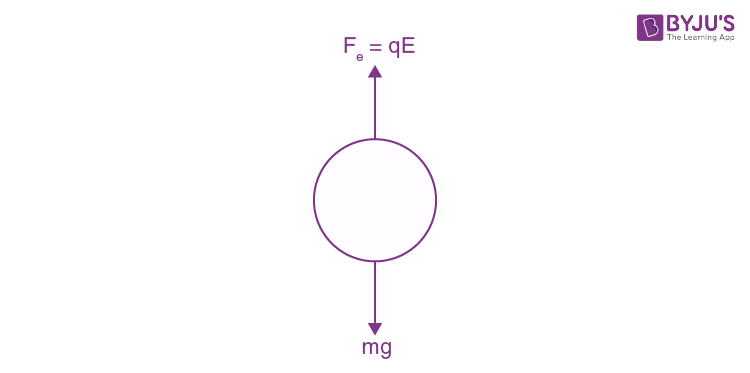Fe = qE = (ne)E

Fe = mg

(ne)E = mg

$$\begin{array}{l}\begin{array}{l} \mathrm{n}=\frac{m g}{e E}=\frac{\rho \frac{4}{3} \pi R^{3} \times g}{e E} \\ \mathrm{n}=\frac{3000 \times \frac{4}{3} \times 3.14 \times 8 \times 10^{-9} \times 9.8}{1.6 \times 10^{-19} \times 3.55 \times 10^{5}} \\ \mathrm{n}=\frac{984704 \times 10^{5}}{5.68}=1.73 \times 10^{10} \end{array}\end{array}$$

n = 1.73 ×1010

Question 12. The time period of a satellite in a circular orbit of radius R is T. The period of another satellite in a circular orbit of radius 9R is :

a. 3T
b. 9 T
c. 27 T
d. 12T

T2∝ R3

$$\begin{array}{l}\frac{T_{1}}{T_{2}}=\frac{R_{1}}{R_{2}}^{3/2}\end{array}$$
$$\begin{array}{l}\frac{T_{1}}{T_{2}}=\frac{R_{1}}{9R}^{3/2}\end{array}$$
$$\begin{array}{l}\frac{T}{T_{2}}=\frac{1}{27}\end{array}$$

T2 = 27T

Question 13. A loop of flexible wire of irregular shape carrying current is placed in an external magnetic field. Identify the effect of the field on the wire

a. Loop assumes a circular shape with its plane parallel to field
b. Shape of the loop remains unchanged
c. Wire gets stretched to become straight
d. Loop assumes circular shape with its plane normal to the field

Force on each small wire segment be along radially outward and equal so, it will take the shape of a circle and parallel to the field.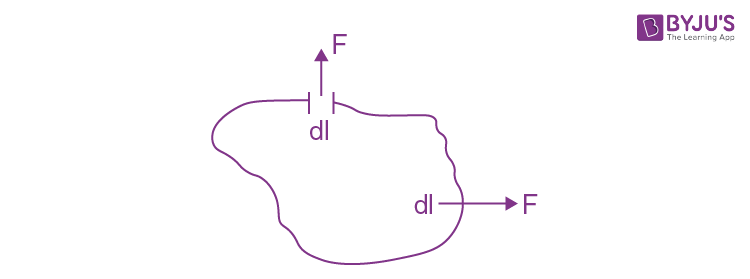Question 14. In Young’s double-slit arrangement, slits are separated by a gap of 0.5 mm, and the screen is placed at a distance of 0.5 m from them. The distance between the first and the third bright fringe formed when the slits are illuminated by monochromatic light of 5890 Å is:

a. 1178 × 10-6m
b. 1178 × 10-9m
c. 5890 × 10-7m
d. 1178× 10-12m

D = 0.5 m

d = 0.5 mm

λ = 5890Å

Distance between first and third bright fringe = 2β = 2λD/d

= 2 × 5890 × 10-10 × 0.5/(0.5×10-3)

= 1178 × 10-6 m

Question 15. Match List – I with List – II

 List – I List – II (a) 10 km height over earth’s surface (i) Thermosphere (b) 70 km height over earth’s surface (ii) Mesosphere (c) 180 km height over earth’s surface (iii) stratosphere (d) 270 km height over earth’s surface (iv) Troposphere

a. (a) – (ii), (b) – (i), (c) – (iv), (d) – (iii)
b. (a) – (iv), (b) – (iii), (c) – (ii), (d) – (i)
c. (a) – (iii), (b) – (ii), (c) – (i), (d) – (iv)
d. (a) – (i), (b) – (iv), (c) – (iii), (d) – (ii)

Troposphere

The troposphere starts at the Earth’s surface and extends 8 to 14.5 kilometres high (6 to 9 miles).Stratosphere

The stratosphere starts just above the troposphere and extends to 50 kilometres (31 miles) high.

Mesosphere

The mesosphere starts just above the stratosphere and extends to 85 kilometres (53 miles) high.

Thermosphere

The Thermosphere starts just above the mesosphere and extends to 600 kilometres (372 miles) high.

Ionosphere

The ionosphere is an abundant layer of electrons and ionized atoms and molecules that stretches from about 48 kilometres (30 miles) above the surface to the edge of space at about 965 km (600 mi).

Exosphere

This is the upper limit of our atmosphere. It extends from the top of the thermosphere up to 10000 km (6200mi).

Question 16. A constant power delivering machine has towed a box, which was initially at rest, along a horizontal straight line. The distance moved by the box in time ‘t’ is proportional to:

a. t
b. t3/2
c. t1/2
d. t2/3

$$\begin{array}{l}\vec{P}=\vec{F}.\vec{V}=\left | \vec{F} \right |\left | \vec{V} \right |cos\theta\end{array}$$

As force and velocity have the same direction the angle between

$$\begin{array}{l}\vec{F}\end{array}$$
and
$$\begin{array}{l}\vec{V}\end{array}$$
is 00.

$$\begin{array}{l}P = \frac{mVdV}{dt}\end{array}$$
$$\begin{array}{l}\int_{0}^{t}Pdt = m\int_{0}^{V}VdV\end{array}$$
$$\begin{array}{l}Pt= \frac{mV^{2}}{2}\end{array}$$
$$\begin{array}{l}V= \sqrt{\frac{2Pt}{m}}\end{array}$$
$$\begin{array}{l}\frac{dx}{dt}=\sqrt{\frac{2Pt}{m}}\end{array}$$
$$\begin{array}{l}\int dx = \int \sqrt{\frac{2Pt}{m}}dt\end{array}$$

x∝t3/2

Question 17. A thin circular ring of mass M and radius r is rotating about its axis with an angular speed ω. Two particles having mass m each are now attached at diametrically opposite points. The angular speed of the ring will become:

a.

$$\begin{array}{l}\omega \frac{M}{M+2m}\end{array}$$

b.
$$\begin{array}{l}\omega \frac{M}{M+m}\end{array}$$

c.
$$\begin{array}{l}\omega \frac{M+2m}{M}\end{array}$$

d.
$$\begin{array}{l}\omega \frac{M-2m}{M+2m}\end{array}$$

τnet = 0, so angular momentum is conserved

By angular momentum conservation

Iiωi = Ifωf

(MR2)ω = (MR2 + 2mR2) ωf

$$\begin{array}{l}\omega _{f}=\frac{(MR^{2}\omega )}{MR^{2}+2mR^{2}}=\frac{M\omega }{M+2m}\end{array}$$
$$\begin{array}{l}\omega _{f}=\frac{M\omega }{M+2m}\end{array}$$

Question 18. Imagine that the electron in a hydrogen atom is replaced by a muon (μ). The mass of a muon particle is 207 times that of an electron and the charge is equal to the charge of an electron. The ionization potential of this hydrogen atom will be:

a. 27.2 eV
b. 331.2 eV
c. 13.6 eV
d. 2815.2 eV

E∝ 1/r

r ∝ 1/m

E ∝ m

Ionization potential = 13.6(massμ)(masse) eV

= 13.6 × 207 eV = 2815.2 eV

Question 19. The P-V diagram of a diatomic ideal gas system going under cyclic process as shown in the figure. The work done during an adiabatic process CD is (use γ = 1.4):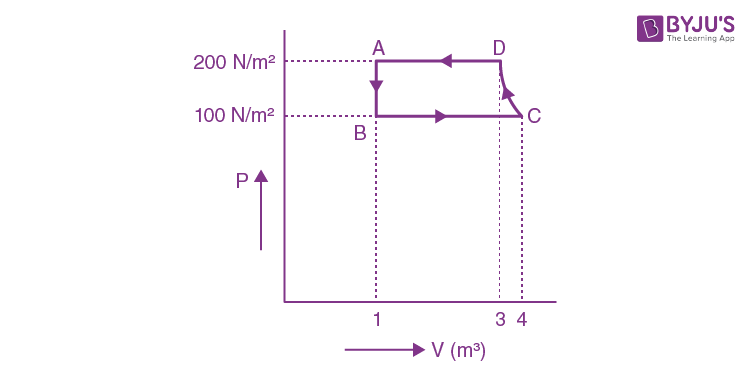a. 200 J
b. –500 J
c. – 400 J
d. 400 J

Work done for the adiabatic process is;

$$\begin{array}{l}W_{CD}=\frac{nR\Delta T}{1-\gamma }=\frac{p_{f}v_{f}-p_{i}v_{i}}{1-\gamma }=\frac{200\times 3-4\times 100}{1-1.4}\end{array}$$
$$\begin{array}{l}W_{CD}=\frac{600-400}{-0.4}=\frac{-200}{0.4}=-500 J\end{array}$$
$$\begin{array}{l}W_{CD}=-500 J\end{array}$$

Question 20. The position, velocity and acceleration of a particle moving with constant acceleration can be represented by: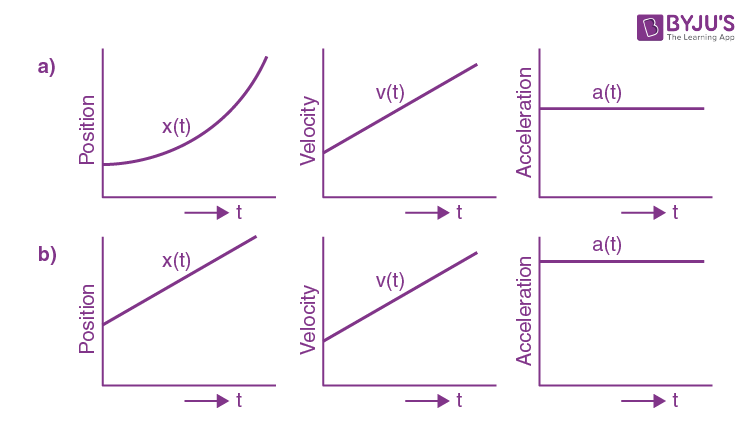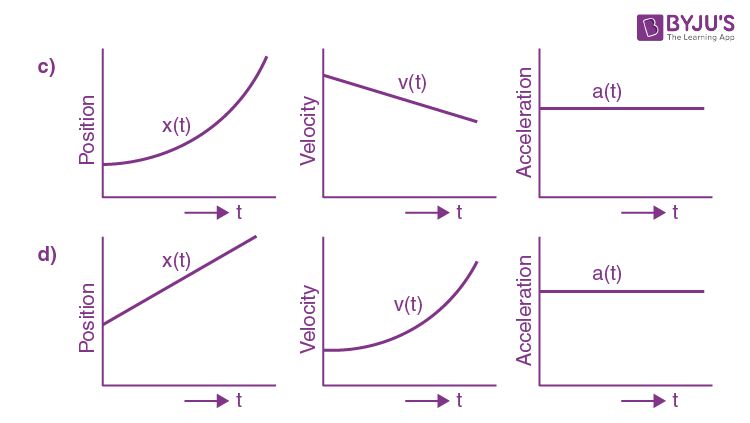Acceleration is constant

a = constant

v ∝ t (straight-line graph)

x ∝ t2 (parabolic graph)

SECTION – B

Question 21. As shown in the figure, a particle of mass 10 kg is placed at point A. When the particle is slightly displaced to its right, it starts moving and reaches point B. The speed of the particle at B is x m/s. (Take g = 10 m/s2) The value of ‘x’ to the nearest integer is _______.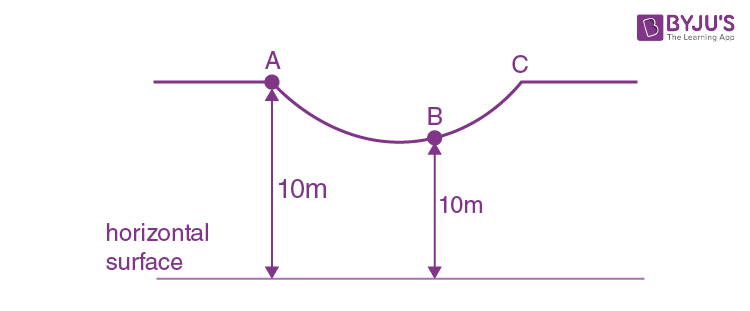Take horizontal surface as reference level for gravitational potential energy

By energy conservation

Ki + Ui= Kf + Uf

0 + 10 × 10 × 10 = (½)×10×vB2+10×10×5

1000 = 5vB2+500

vB2=500/5=100

vB = 10 m/s

x = 10

Question 22. A parallel plate capacitor has a plate area of 100 m2 and plate separation of 10 m. The space between the plates is filled up to a thickness of 5 m with a material of dielectric constant 10. The resultant capacitance of the system is ‘x’ pF. The value of ε0 = 8.85 × 10-12 F.m-1. The value of ‘x’ to the nearest integer is _______.

Area = 100 m2

Separation (d) = 10 m

Thickness = 5 m

Dielectric constant (K) = 10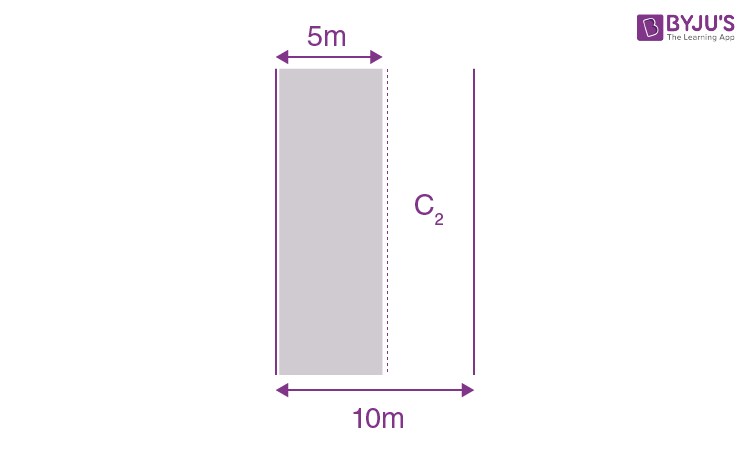$$\begin{array}{l}C_{1}=\frac{KA\varepsilon _{0}}{d}\end{array}$$
$$\begin{array}{l}C_{2}=\frac{A\varepsilon _{0}}{d}\end{array}$$
$$\begin{array}{l}C_{eq}=\frac{C_{1}C_{2}}{C_{1}+C_{2}}=\frac{\frac{KA\varepsilon _{0}}{d}\times \frac{A\varepsilon _{0}}{d}}{\frac{KA\varepsilon _{0}}{d}+\ \frac{A\varepsilon _{0}}{d}}\end{array}$$
$$\begin{array}{l}C_{eq}=\frac{KA^{2}\varepsilon _{0}^{2}}{d^{2}}\times \frac{d}{A\varepsilon _{0}(1+K)}\end{array}$$
$$\begin{array}{l}C_{eq}=\frac{KA\varepsilon _{0}}{d(1+K)}= \frac{10\times 100\times 8.85\times 10^{-12}}{5(1+10)}\end{array}$$
$$\begin{array}{l}C_{eq}= \frac{8.85\times 10^{-19}}{55}=0.1609090 \times 10^{-9} F\end{array}$$

Ceq = 160.90×10-12 F

Ceq = 161 pF

Question 23. An NPN transistor operates as a common emitter amplifier with a power gain of 106. The input circuit resistance is 100 Ω and the output load resistance is 10 kΩ. The common-emitter current gain ‘β’ will be ______. (Round off to the nearest integer)

Power gain = 106

Input resistance = 100 Ω

Output load resistance = 10 kΩ

Power gain = β2 x (10 x 103)/100

β2 = 104 ⇒ β = 100

Question 24. The voltage across the 10 Ω resistor in the given circuit is x volt.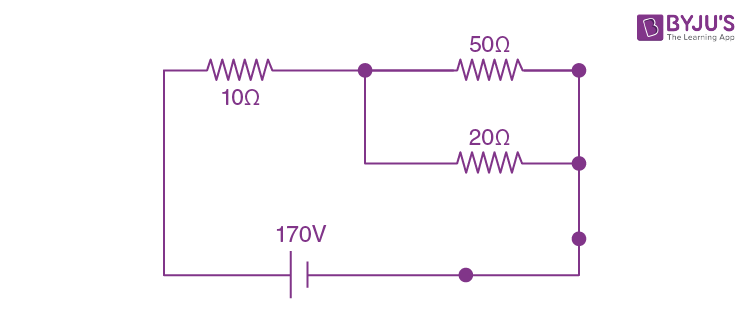The value of ‘x’ to the nearest integer is_________.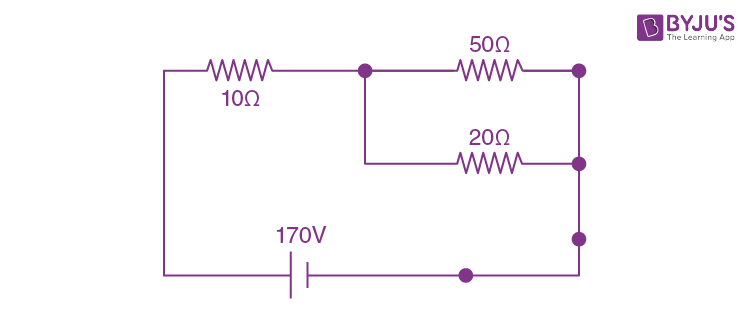Equivalent resistance of 50 Ω and 20 Ω

$$\begin{array}{l}R_{eq}=\frac{50\times 20}{50+20}\end{array}$$
$$\begin{array}{l}R_{eq}=\frac{1000}{70}= \frac{100}{7}\Omega\end{array}$$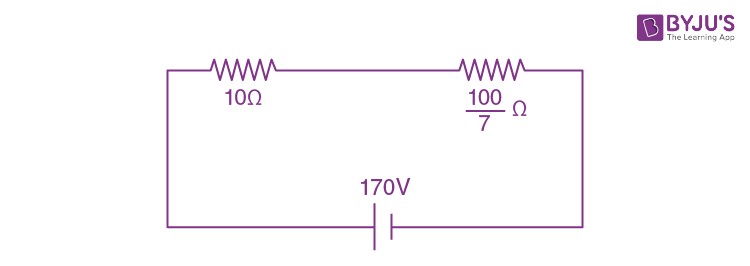Req = 10 + 100/7=170/7 Ω

I = V/R=170/(170/7)

I = 7 A

Potential Across 10 Ω resistor

V10 = IR = 7 × 10 = 70 V

V10 = 70 V

Question 25. Two separate wires A and B are stretched by 2 mm and 4 mm respectively, when they are subjected to a force of 2 N. Assume that both the wires are made up of the same material and the radius of wire B is 4 times that of the radius of wire A. The length of the wires A and B are in the ratio of a:b, Then a/b can be expressed as 1/x where x is.

FA = FB

rB = 4rA

Δla = 2 mm

ΔlB = 4 mm

Y=stress/strain

=F/A / Δl/l

Δl/l=F/AY

$$\begin{array}{l}I= \frac{AY\Delta l}{F}\end{array}$$
$$\begin{array}{l}\frac{l_{a}}{l_{b}}=\frac{\pi r^{2}_{a}\times Y\times \Delta l_{a}\times F}{\pi r^{2}_{b}\times Y\times \Delta l_{b}\times F}\end{array}$$
$$\begin{array}{l}\frac{l_{a}}{l_{b}}=\frac{ r^{2}_{a}\times \Delta l_{a}}{r^{2}_{b}\times \Delta l_{b}}=\frac{r^{2}_{a}\times 2}{(4r_{a})^{2}\times 4}=\frac{r_{a}^{2}}{16r_{a}^{2}\times 2}\end{array}$$
$$\begin{array}{l}\frac{l_{a}}{l_{b}}= \frac{1}{32}\end{array}$$

X = 32

Question 26. A bullet of mass 0.1 kg is fired on a wooden block to pierce through it, but it stops after moving a distance of 50 cm into it. If the velocity of the bullet before hitting the wood is 10 m/s and it slows down with uniform deceleration, then the magnitude of effective retarding force on the bullet is ‘x’ N. The value of ‘x’ to the nearest integer is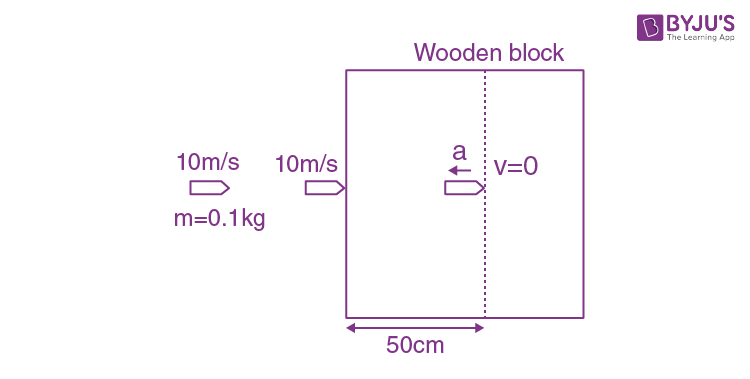Mbullet = 0.1kg

v2 = 102 + 2(-a) × 0.5

0= (10)2 – 2a × (0.5)

Retardation (a) = 100/(2×0.5)=100m/s2

Retarding force (F) = ma = 0.1 × 100

F = 10N

Question 27. A ball of mass 10 kg moving with a velocity 10√ 3 m/s along the x-axis, hits another ball of mass 20 kg which is at rest. After the collision, the first ball comes to rest while the second ball disintegrates into two equal pieces. One-piece starts moving along the y-axis with a speed of 10 m/s. The second piece starts moving at an angle of 30o with respect to the x-axis. The velocity of the ball moving at 30o with x-axis is x m/s.The configuration of pieces after collision is shown in the figure below The value of x to the nearest integer is ______.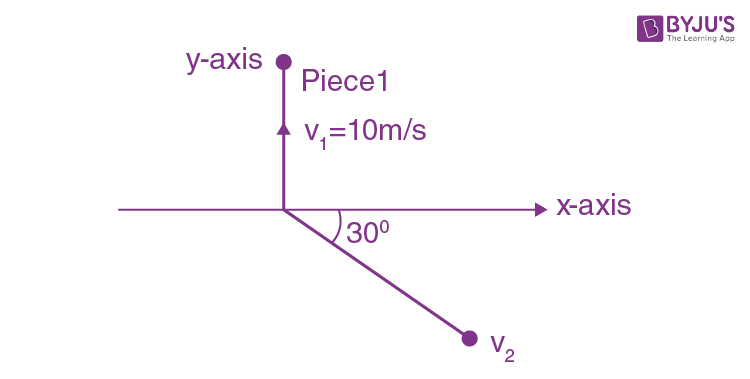Before collision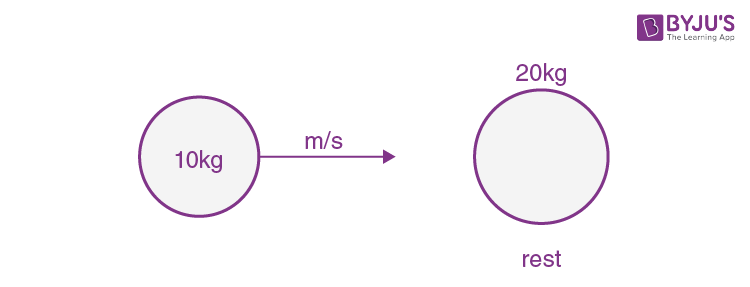In x – direction

Pi = 10×10√ 3 + 20 × 0

Pi = 100 √ 3 kg m/s

After collision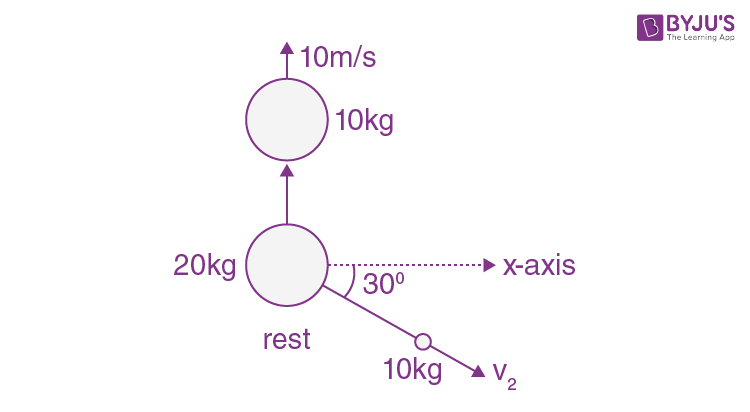In x – direction

Pf = 10 × v2 cos30o

In y direction

Pf = 10 × 10 – 10 v2 sin 30o

Pf = 100 – 5v2

Apply momentum conservation in x-direction

Pi = pf

100√ 3=(10√ 3/2)v2

v2 = 20 m/s

So, answer is v2 = 20 m/s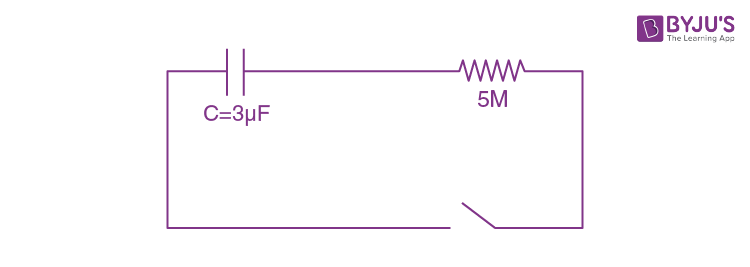Question 28: The circuit shown in the figure consists of a charged capacitor of capacity 3 µF and a charge of 30 µC. At time t = 0, when the key is closed, the value of current flowing through the 5MΩ resistor is ‘x’ µA. The value of ‘x’ to the nearest integer is _______.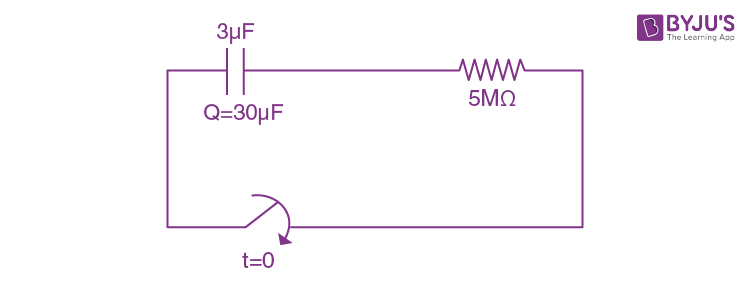$$\begin{array}{l}Q = Q_{0}e^{-t/RC}\end{array}$$
$$\begin{array}{l}I = \frac{Q_{0}}{RC}e^{-t/RC}\end{array}$$

Q0 = 30×10–6 C

C = 3×10–6 F

at t = 0,

$$\begin{array}{l}I = \frac{Q_{0}}{RC}e^{-0/RC}\end{array}$$

I = Q/RC = 30/(5 x 106 x 3) = 2 x 10-6 A

I = 2 μA

Question 29. A person is swimming with a speed of 10 m/s at an angle of 120o with the flow and reaches to a point directly opposite on the other side of the river. Then the speed of the flow is ‘x’ m/s. The value of ‘x’ to the nearest integer is _____.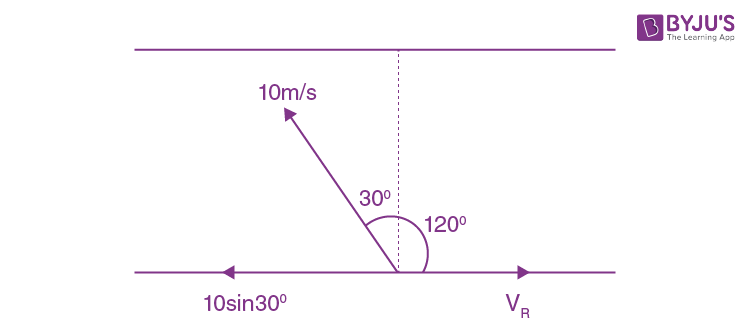Velocity along the river

VR = 10 sin 300

VR = 10/2=5m/s

VR = 5 m/s

Question 30. A particle performs simple harmonic motion with a period of 2 second. The time taken by the particle to cover a displacement equal to half of its amplitude from the mean position is (1/a) s. The value of ‘a’ to the nearest integer is _____.

Time period (T) = 2 sec.

x = A sin (ωt + φ) (φ = 0 at M.P.)

A/2 = A sin(2π/T)t

(2π/T)t=π/6

t = 1/6 s

a = 6